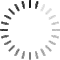# Pointer Arithmetic

0
0

Hello Sir,

I have a small question :
I want to find the size of structure, “node” with out sizeof operator.
In the code snippet mentioned below, the address of the 2 elements of array is printed and the offset is also correct, according to the size of the struct node.
However, when I subtract and print the offset value, the value is 1 instead of the actual size.

But it is correct in the second approach.
Can you please tell me what the issue in first case??

Code Snippet :

struct node {
int data;
};

int main (){

struct node NodeAry, *ptrNode = 0;
printf (“Address of nodes is := %p %p\n”, &NodeAry,&NodeAry ); //Prints correct difference
int size_of = (&NodeAry – &NodeAry); //<- This offset can be printed directly.
printf (“sizeof node is := %d\n”,  size_of);  //Wrong Size

*ptrNode++;
printf (“Sizeof Struct using ptr++ := %d\n”, ptrNode); //Correct Size

return 0;
}

Sample Output:
Address of nodes is :=  0028ff34 0028ff2c
sizeof node is := 1   // <- This is wrong output.
Sizeof Struct using ptr++ := 8

I executed this in windows, using codeblocks and using gcc compiler.

Thanks in advance for the time took to go through this.

Thanks,

Jay

• You must to post comments
0
0

Dear S,

When you do   (   &NodeAry – &NodeAry  ),  you are subtracting a pointer from another of the same type.  The C compiler automatically divides the difference by the size of the item being pointed to.  Hence you will always get value of 1  for any type of array for the expression above.  This is simple to understand if you look at the following code snippet.

int x;

SomeType * p, * q;

q = p + x;    // Note that the compiler adds  ( x * sizeof (*p) ) to pointer p value to get q.

Hence,  when you use the expression  ( q – p ),  you can see that the result must be   x   by simple transposition.  This can be obtained only by divided the address difference by the sizeof (*p).

M.L.S. Shastry

• You must to post comments
0
0

Hi Sir,

Thanks for the explanatory answer.

In fact I was looking at the same matter as well.

I changed the line to :    int size_of = ((int)&NodeAry – (int)&NodeAry);

And this gave me the expected result.

Thanks,

Jay

• You must to post comments
Showing 2 results